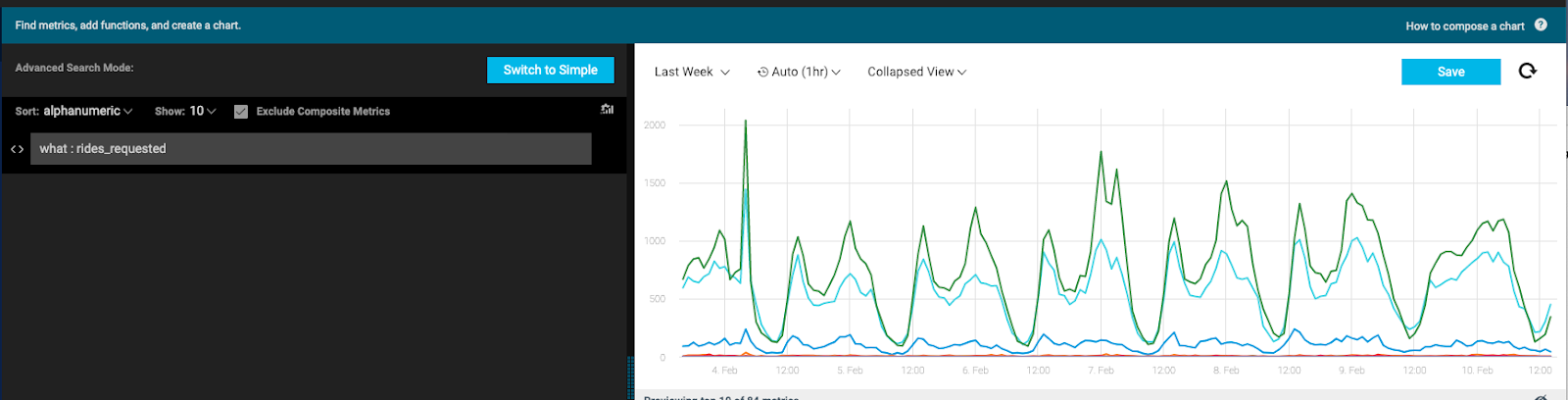StdSeries

 Description Calculates and recalculates the running average of the series and its standard deviation at any given point. Input Time Series – multiple (Single or multiple time series.) Parameters None Output Std Time Series – single Available in Alerts + Composite + Dashboards

USAGE EXAMPLE

In order to best apply the stdSeries function we have to understand what is a standard deviation:

Standard deviation is a number used to tell how measurements for a group are spread out from the average (mean), or expected value. A low standard deviation means that most of the numbers are very close to the average. A high standard deviation means that the numbers are spread out. In other words, it provides the amount of variance (of the data) around the average for each point.

For example, lets say you're measuring the number of rides requested:And then apply the stdSeries function:You can catch noise change where the baseline won't necessarily catch the anomaly.